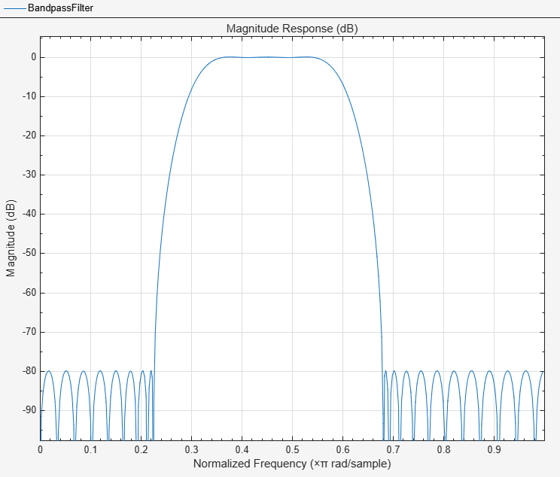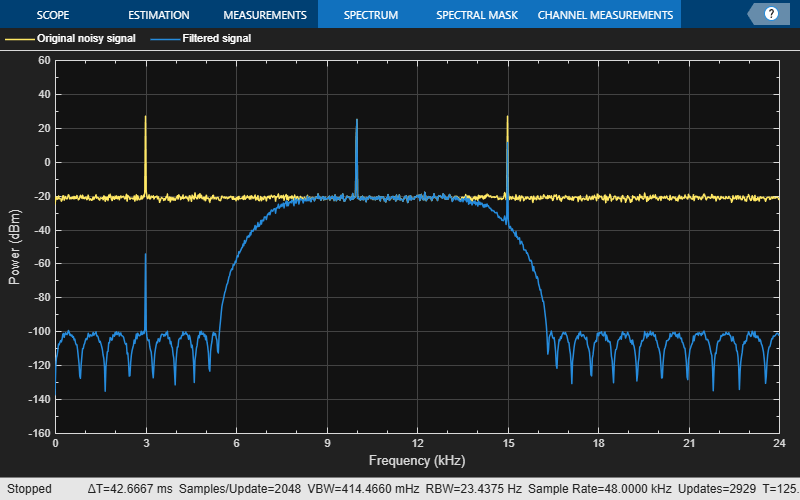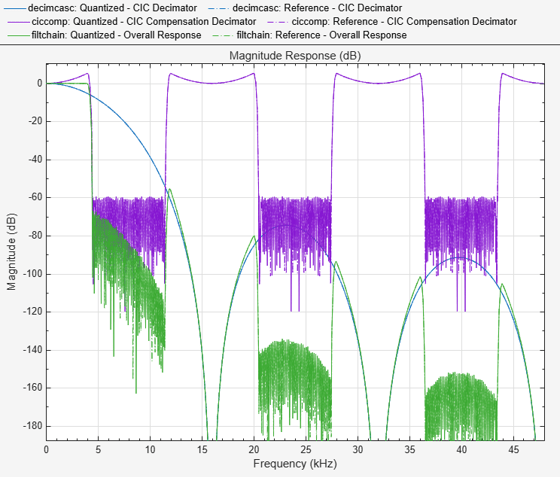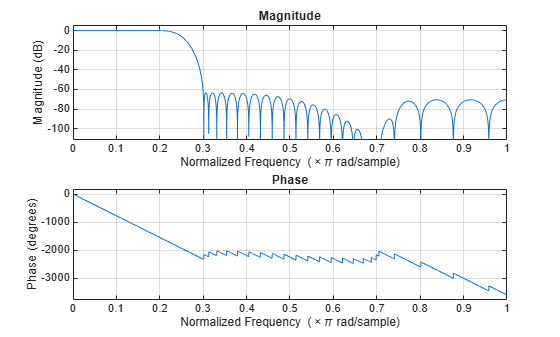Documentation

### This is machine translation

Mouseover text to see original. Click the button below to return to the English version of the page.

Create a cascade of filter System objects

## Description

The `dsp.FilterCascade` object creates a multistage System object™ that enables cascading of filter System objects, delays, and scalar gains. This object operates similar to the `cascade` function. However, the `cascade` function does not support delay as a filter stage.

You can pass the `dsp.FilterCascade` System object as a stage to another `dsp.FilterCascade` System object. You can also pass `dsp.FilterCascade` System object as an input to the `cascade` function.

When you call the object, the size, data type, and complexity of the input signal must be supported by all of the stages in the filter cascade. This object supports variable-size signals if the stages within support variable-size signals.

To filter a signal with a cascade of filters:

1. Create the `dsp.FilterCascade` object and set its properties.

2. Call the object with arguments, as if it were a function.

Alternatively, you can generate a MATLAB® function from the filter cascade object, and call that function to filter a signal. The generated function supports C/C++ code generation. See `generateFilteringCode`.

## Creation

### Syntax

``FC=dsp.FilterCascade``
``FC=dsp.FilterCascade(filt1,filt2,...)``

### Description

````FC=dsp.FilterCascade` returns a System object, `FC`. This System object has a single stage, the `dsp.FIRFilter` object with default properties.```

example

````FC=dsp.FilterCascade(filt1,filt2,...)` returns a multistage System object, `FC`, with the first stage set to `filt1`, the second stage set to `filt2`, and so on. Each stage can be a filter System object , a `dsp.FilterCascade` System object, a `dsp.Delay` System object, or a scalar gain value.For example,lpFilt = dsp.LowpassFilter('StopbandFrequency',15000,... 'PassbandFrequency',12000); hpFilt = dsp.HighpassFilter('StopbandFrequency',5000,... 'PassbandFrequency',8000); gain = 2; bpFilt = dsp.FilterCascade(lpFilt,hpFilt,2); ```

## Properties

expand all

Unless otherwise indicated, properties are nontunable, which means you cannot change their values after calling the object. Objects lock when you call them, and the `release` function unlocks them.

If a property is tunable, you can change its value at any time.

Filter stage, specified as a filter System object, delay System object, or a scalar gain value. To see which System objects you can add to a filter cascade, use:

`dsp.FilterCascade.helpSupportedSystemObjects`
You can modify an existing stage by modifying the associated property. For example:
```FC = dsp.FilterCascade(dsp.FIRFilter,5) FC = dsp.FilterCascade with properties: Stage1: [1×1 dsp.FIRFilter] Stage2: 5 K>> FC.Stage2 = dsp.FIRDecimator FC = dsp.FilterCascade with properties: Stage1: [1×1 dsp.FIRFilter] Stage2: [1×1 dsp.FIRDecimator]```
To change the number of stages in a cascade, use the `addStage` and `removeStage` functions.

## Usage

For versions earlier than R2016b, use the `step` function to run the System object algorithm. The arguments to `step` are the object you created, followed by the arguments shown in this section.

For example, `y = step(obj,x)` and `y = obj(x)` perform equivalent operations.

### Syntax

``y=FC(x)``

### Description

example

````y=FC(x)` filters the input signal `x` using the filter cascade defined in `FC`, and returns the filtered output, `y`. The size, data type, and complexity of the input signal must be supported by all of the stages in the filter cascade. This object supports variable-size signals if the stages within support variable-size signals.```

### Input Arguments

expand all

Data input, specified as a vector or a matrix. When the input is a matrix, each column of the matrix represents an independent data channel.

Data Types: `single` | `double`
Complex Number Support: Yes

### Output Arguments

expand all

Filtered output, returned as a vector or a matrix. The size, data type, and complexity of the output signal matches that of the input signal.

Data Types: `double` | `single`
Complex Number Support: Yes

## Object Functions

To use an object function, specify the System object as the first input argument. For example, to release system resources of a System object named `obj`, use this syntax:

`release(obj)`

expand all

 `addStage` Add new filter stage to cascade `generateFilteringCode` Generate MATLAB code for a filter cascade `getNumStages` Get number of stages in filter cascade `releaseStages` Release the locked state of all stages in the cascade `removeStage` Remove stage from filter cascade
 `step` Run System object algorithm `release` Release resources and allow changes to System object property values and input characteristics `reset` Reset internal states of System object

## Examples

expand all

Note: This example runs only in R2016b or later. If you are using an earlier release, replace each call to the function with the equivalent `step` syntax. For example, `myObject(x)` becomes `step(myObject,x)`.

Design a bandpass filter by cascading:

• A highpass filter with a stopband frequency of 5000 Hz and a passband frequency of 8000 Hz.

• A lowpass filter with a passband frequency of 12,000 Hz and a stopband frequency of 15,000 Hz.

Visualize the frequency response using `fvtool`.

```lpFilt = dsp.LowpassFilter('StopbandFrequency',15000,... 'PassbandFrequency',12000); hpFilt = dsp.HighpassFilter('StopbandFrequency',5000,... 'PassbandFrequency',8000); bpFilt = dsp.FilterCascade(lpFilt,hpFilt); fvtool(bpFilt); legend('Bandpass Filter'); ```Pass a noisy sine wave as the input to the bandpass filter. The input is a sum of three sine waves with frequencies at 3 kHz, 10 kHz, and 15 kHz. The sampling frequency is 48 kHz. View the input and the filtered output on a spectrum analyzer.

```Sine1 = dsp.SineWave('Frequency',3e3,'SampleRate',48e3,'SamplesPerFrame',6000); Sine2 = dsp.SineWave('Frequency',10e3,'SampleRate',48e3,'SamplesPerFrame',6000); Sine3 = dsp.SineWave('Frequency',15e3,'SampleRate',48e3,'SamplesPerFrame',6000); SpecAna = dsp.SpectrumAnalyzer('PlotAsTwoSidedSpectrum',false, ... 'SampleRate',Sine1.SampleRate, ... 'NumInputPorts',2,... 'ShowLegend',true, ... 'YLimits',[-160,60]); SpecAna.ChannelNames = {'Original noisy signal','Filtered signal'}; for i = 1 : 1000 x = Sine1()+Sine2()+Sine3()+0.1.*randn(Sine1.SamplesPerFrame,1); y = bpFilt(x); SpecAna(x,y); end release(SpecAna) ```The tones at 3 kHz and 15 kHz are attenuated, and the tone at 10 kHz is preserved by the bandpass filter.

Create a CIC decimator. Cascade the decimator with a gain.

```cicdecim = dsp.CICDecimator('DecimationFactor', 6, ... 'NumSections', 6); decimcasc = dsp.FilterCascade(cicdecim, 1/gain(cicdecim));```

Design a compensation decimator and cascade it with the filter cascade, `decimcasc`.

```fs = 16e3; % Sampling frequency of input of compensation decimator fPass = 4e3; % Passband frequency fStop = 4.5e3; % Stopband frequency ciccomp = dsp.CICCompensationDecimator(cicdecim, ... 'DecimationFactor', 2, ... 'PassbandFrequency', fPass, ... 'StopbandFrequency', fStop, ... 'SampleRate', fs); filtchain = dsp.FilterCascade(decimcasc, ciccomp);```

```f = fvtool(decimcasc, ciccomp, filtchain, 'Fs',[fs*6,fs,fs*6],... 'Arithmetic', 'fixed'); legend(f,'CIC Decimator','CIC Compensation Decimator', ... 'Overall Response');```The CIC compensation decimator has an inherent gain, `gain(cicdecim)`. By cascading with a factor of `1/gain(cicdecim)`, the filter cascade compensates for this gain.

Design a two-stage decimator with a 100 Hz transition width, a 2 kHz sampling frequency, and 60 dB attenuation in the stopband. The decimator needs to downsample by a factor of 4.

```decimSpec = fdesign.decimator(4, 'Nyquist', 4, 'Tw,Ast', 100,60,2000); filtCasc = design(decimSpec, 'multistage', 'SystemObject', true);```

Verify your design using `fvtool`.

` info(filtCasc)`
```ans = 'Discrete-Time Filter Cascade ---------------------------- Number of stages: 2 Stage1: dsp.FIRDecimator ------- Discrete-Time FIR Multirate Filter (real) ----------------------------------------- Filter Structure : Direct-Form FIR Polyphase Decimator Decimation Factor : 2 Polyphase Length : 10 Filter Length : 19 Stable : Yes Linear Phase : Yes (Type 1) Arithmetic : double Stage2: dsp.FIRDecimator ------- Discrete-Time FIR Multirate Filter (real) ----------------------------------------- Filter Structure : Direct-Form FIR Polyphase Decimator Decimation Factor : 2 Polyphase Length : 18 Filter Length : 35 Stable : Yes Linear Phase : Yes (Type 1) Arithmetic : double ' ```
` fvtool(filtCasc)`Generate code to filter data using this design. The code is saved in a file called `stepDecimator.m` in the current directory.

` generateFilteringCode(filtCasc, 'stepDecimator');`

The `stepDecimator` function creates a filter cascade and calls the `step` method on each stage.

` type stepDecimator`
```function y = stepDecimator(x) %STEPDECIMATOR Construct filter cascade and process each stage % MATLAB Code % Generated by MATLAB(R) 9.6 and DSP System Toolbox 9.8. % Generated on: 13-Apr-2019 02:24:03 % To generate C/C++ code from this function use the codegen command. % Type 'help codegen' for more information. %#codegen %% Construction persistent filter1 filter2 if isempty(filter1) filter1 = dsp.FIRDecimator( ... 'Numerator', [0.0021878514650437845 0 -0.010189095418136306 0 0.031140395225498115 0 -0.082785931644222821 0 0.30979571849010851 0.5 0.30979571849010851 0 -0.082785931644222821 0 0.031140395225498115 0 -0.010189095418136306 0 0.0021878514650437845]); filter2 = dsp.FIRDecimator( ... 'Numerator', [0.0011555011750488237 0 -0.0027482166351233102 0 0.0057681982289523072 0 -0.010736374060960912 0 0.018592020073668478 0 -0.031093723586671229 0 0.052603914610235683 0 -0.099130756073130377 0 0.31592697826202448 0.5 0.31592697826202448 0 -0.099130756073130377 0 0.052603914610235683 0 -0.031093723586671229 0 0.018592020073668478 0 -0.010736374060960912 0 0.0057681982289523072 0 -0.0027482166351233102 0 0.0011555011750488237]); end %% Process y1 = filter1( x ); y = filter2( y1); ```